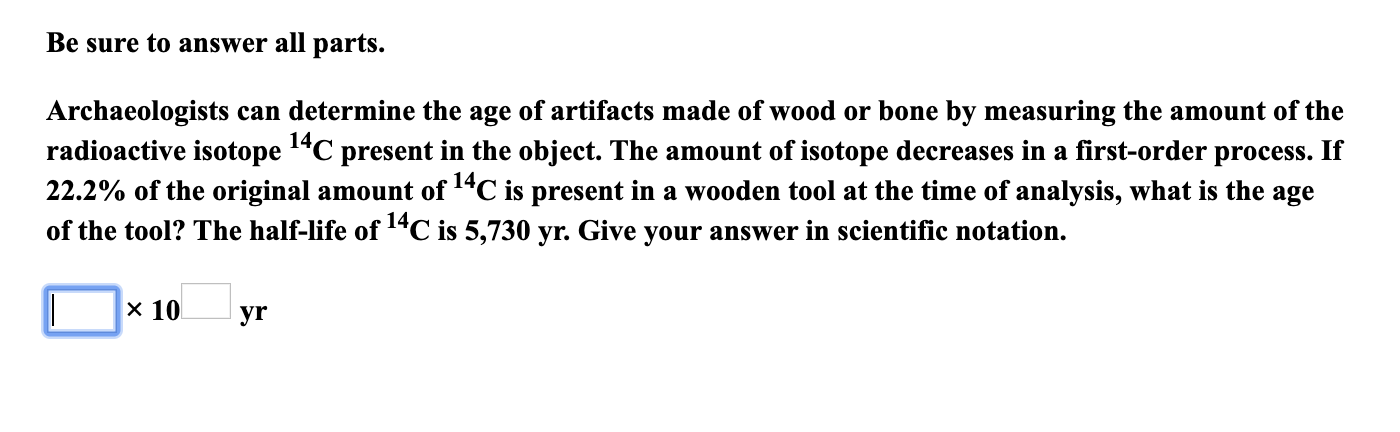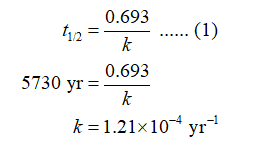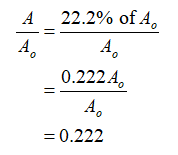# Be sure to answer all parts.Archaeologists can determine the age of artifacts made of wood or bone by measuring the amount of theradioactive isotope 1"C present in the object. The amount of isotope decreases in a first-order process. If22.2% of the original amount of 14C is present in a wooden tool at the time of analysis, what is the ageof the tool? The half-life of 14C is 5,730 yr. Give your answer in scientific notation.14,x 10yr

Question
17 viewshelp_outlineImage TranscriptioncloseBe sure to answer all parts. Archaeologists can determine the age of artifacts made of wood or bone by measuring the amount of the radioactive isotope 1"C present in the object. The amount of isotope decreases in a first-order process. If 22.2% of the original amount of 14C is present in a wooden tool at the time of analysis, what is the age of the tool? The half-life of 14C is 5,730 yr. Give your answer in scientific notation. 14, x 10 yr fullscreen
check_circle

Step 1

The rate constant (k) of the given first-order process is calculated using equation (1) in which t1/2 is the half life of carbon-14.Step 2

The ratio of the amount present at the time of analysis (A) to the original amount (Ao) is calculated as follows:...

### Want to see the full answer?

See Solution

#### Want to see this answer and more?

Solutions are written by subject experts who are available 24/7. Questions are typically answered within 1 hour.*

See Solution
*Response times may vary by subject and question.
Tagged in

### Chemistry